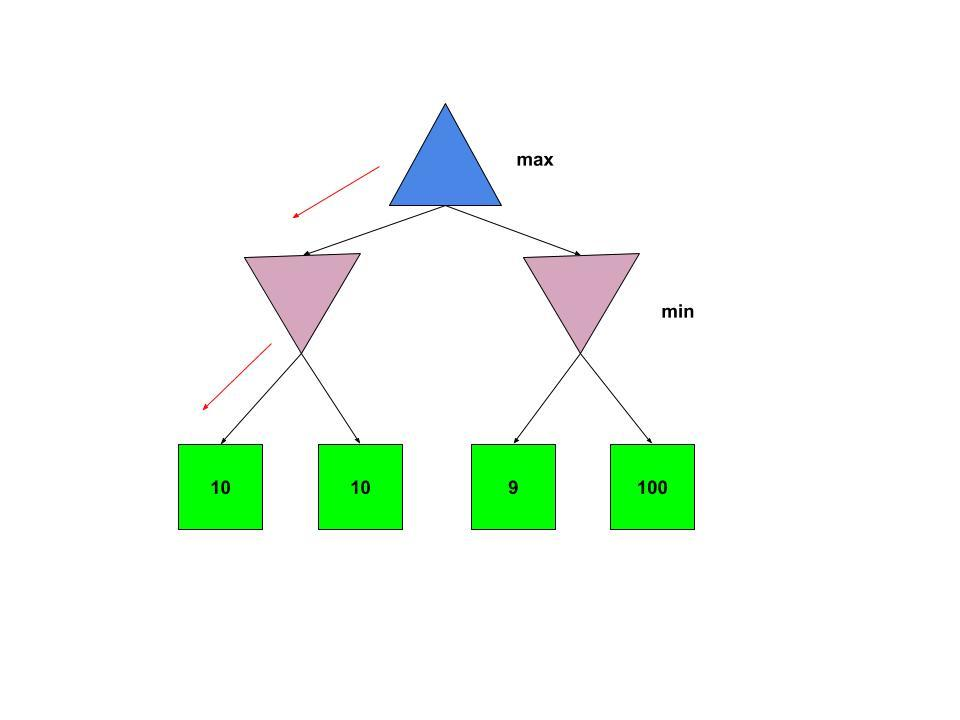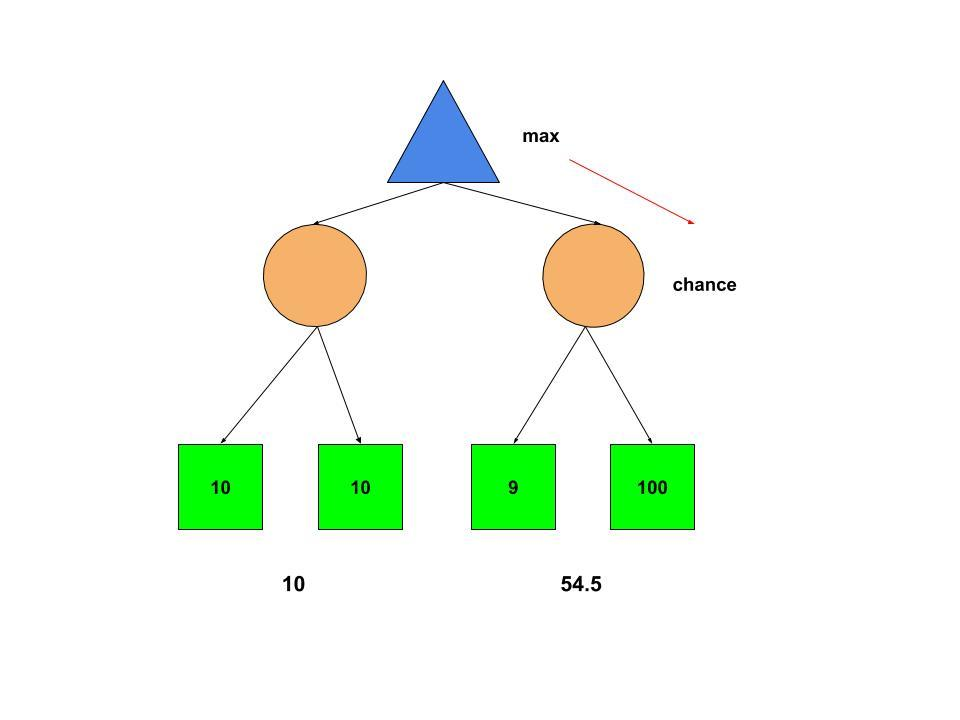# Expectimax Algorithm in Game Theory

The Expectimax search algorithm is a game theory algorithm used to maximize the expected utility. It is a variation of the Minimax algorithm. While Minimax assumes that the adversary(the minimizer) plays optimally, the Expectimax doesn’t. This is useful for modelling environments where adversary agents are not optimal, or their actions are based on chance.

Expectimax vs Minimax
Consider the below Minimax tree:As we know that the adversary agent(minimizer) plays optimally, it makes sense to go to the left. But what if there is a possibility of the minimizer making a mistake(or not playing optimally). Therefore going right might sound more appealing or may result in a better solution.

In the below Expectimax tree, we have replaced minimizer nodes by chance nodes.The Chance nodes take the average of all available utilities giving us the ‘expected utility’. Thus the expected utilities for left and right sub-trees are (10+10)/2=10 and (100+9)/2=54.5. The maximizer node chooses the right sub-tree to maximize the expected utilities.

• Expectimax algorithm helps take advantage of non-optimal opponents.
• Unlike Minimax, Expectimax ‘can take a risk’ and end up in a state with a higher utility as opponents are random(not optimal).

• Expectimax is not optimal. It may lead to the agent losing(ending up in a state with lesser utility)
• Expectimax requires the full search tree to be explored. There is no type of pruning that can be done, as the value of a single unexplored utility can change the expectimax value drastically. Therefore it can be slow.
• It is sensitive to monotonic transformations in utility values.

For minimax, if we have two states S1 and S2, if S1 is better than S2, the magnitudes of the evalaution function values f(S1) and f(S2) don’t matter as along as f(S1)>f(S2).
For expectimax, magnitudes of the evaluation function values matter.

Algorithm: Expectimax can be implemented using recursive algorithm as follows,

1. If the current call is a maximizer node, return the maximum of the state values of the nodes successors.
2. If the current call is a chance node, then return the average of the state values of the nodes successors(assuming all nodes have equal probability). If different nodes have different probabilities the expected utility from there is given by ixipi
3. We call the function recursively until we reach a terminal node(the state with no successors). Then return the utility for that state.

Implementation:

## C++

 `// C++ program to illustrate ` `// Expectimax Algorithm ` ` `  `#include ` `using` `namespace` `std; ` ` `  `// Structure to declare ` `// left and right nodes ` `struct` `Node { ` `    ``int` `value; ` `    ``struct` `Node *left, *right; ` `}; ` ` `  `// Initializing Nodes to NULL ` `Node* newNode(``int` `v) ` `{ ` `    ``Node* temp = ``new` `Node; ` `    ``temp->value = v; ` `    ``temp->left = NULL; ` `    ``temp->right = NULL; ` `    ``return` `temp; ` `} ` ` `  `// Getting expectimax ` `float` `expectimax(Node* node, ``bool` `is_max) ` `{ ` `    ``// Condition for Terminal node ` `    ``if` `(node->left == NULL ` `        ``&& node->right == NULL) { ` `        ``return` `node->value; ` `    ``} ` ` `  `    ``// Maximizer node. Chooses the max from the ` `    ``// left and right sub-trees ` `    ``if` `(is_max) { ` `        ``return` `max( ` `            ``expectimax( ` `                ``node->left, ``false``), ` `            ``expectimax(node->right, ``false``)); ` `    ``} ` ` `  `    ``// Chance node. Returns the average of ` `    ``// the left and right sub-trees ` `    ``else` `{ ` `        ``return` `( ` `                   ``expectimax(node->left, ``true``) ` `                   ``+ expectimax(node->right, ``true``)) ` `               ``/ 2.0; ` `    ``} ` `} ` ` `  `// Driver code ` `int` `main() ` `{ ` `    ``// Non leaf nodes. ` `    ``// If search is limited ` `    ``// to a given depth, ` `    ``// their values are ` `    ``// taken as heuristic value. ` `    ``// But because the entire tree ` `    ``// is searched their ` `    ``// values don't matter ` `    ``Node* root = newNode(0); ` `    ``root->left = newNode(0); ` `    ``root->right = newNode(0); ` ` `  `    ``// Assigning values to Leaf nodes ` `    ``root->left->left = newNode(10); ` `    ``root->left->right = newNode(10); ` `    ``root->right->left = newNode(9); ` `    ``root->right->right = newNode(100); ` ` `  `    ``float` `res = expectimax(root, ``true``); ` `    ``cout << ``"Expectimax value is "`  `<< res << endl; ` `    ``return` `0; ` `} `

## Java

 `// Java program to illustrate ` `// Expectimax Algorithm ` `class` `GFG{ ` `  `  `// Structure to declare ` `// left and right nodes ` `static` `class` `Node { ` `    ``int` `value; ` `    ``Node left, right; ` `}; ` `  `  `// Initializing Nodes to null ` `static` `Node newNode(``int` `v) ` `{ ` `    ``Node temp = ``new` `Node(); ` `    ``temp.value = v; ` `    ``temp.left = ``null``; ` `    ``temp.right = ``null``; ` `    ``return` `temp; ` `} ` `  `  `// Getting expectimax ` `static` `float` `expectimax(Node node, ``boolean` `is_max) ` `{ ` `    ``// Condition for Terminal node ` `    ``if` `(node.left == ``null` `        ``&& node.right == ``null``) { ` `        ``return` `node.value; ` `    ``} ` `  `  `    ``// Maximizer node. Chooses the max from the ` `    ``// left and right sub-trees ` `    ``if` `(is_max) { ` `        ``return` `Math.max( ` `            ``expectimax( ` `                ``node.left, ``false``), ` `            ``expectimax(node.right, ``false``)); ` `    ``} ` `  `  `    ``// Chance node. Returns the average of ` `    ``// the left and right sub-trees ` `    ``else` `{ ` `        ``return` `(``float``) (( ` `                   ``expectimax(node.left, ``true``) ` `                   ``+ expectimax(node.right, ``true``)) ` `               ``/ ``2.0``); ` `    ``} ` `} ` `  `  `// Driver code ` `public` `static` `void` `main(String[] args) ` `{ ` `    ``// Non leaf nodes. ` `    ``// If search is limited ` `    ``// to a given depth, ` `    ``// their values are ` `    ``// taken as heuristic value. ` `    ``// But because the entire tree ` `    ``// is searched their ` `    ``// values don't matter ` `    ``Node root = newNode(``0``); ` `    ``root.left = newNode(``0``); ` `    ``root.right = newNode(``0``); ` `  `  `    ``// Assigning values to Leaf nodes ` `    ``root.left.left = newNode(``10``); ` `    ``root.left.right = newNode(``10``); ` `    ``root.right.left = newNode(``9``); ` `    ``root.right.right = newNode(``100``); ` `  `  `    ``float` `res = expectimax(root, ``true``); ` `    ``System.out.print(``"Expectimax value is "` `+ res +``"\n"``); ` `} ` `} ` ` `  `// This code is contributed by PrinciRaj1992 `

## C#

 `// C# program to illustrate ` `// Expectimax Algorithm ` `using` `System; ` ` `  `class` `GFG{ ` `   `  `// Structure to declare ` `// left and right nodes ` `class` `Node { ` `    ``public` `int` `value; ` `    ``public` `Node left, right; ` `}; ` `   `  `// Initializing Nodes to null ` `static` `Node newNode(``int` `v) ` `{ ` `    ``Node temp = ``new` `Node(); ` `    ``temp.value = v; ` `    ``temp.left = ``null``; ` `    ``temp.right = ``null``; ` `    ``return` `temp; ` `} ` `   `  `// Getting expectimax ` `static` `float` `expectimax(Node node, ``bool` `is_max) ` `{ ` `    ``// Condition for Terminal node ` `    ``if` `(node.left == ``null` `        ``&& node.right == ``null``) { ` `        ``return` `node.value; ` `    ``} ` `   `  `    ``// Maximizer node. Chooses the max from the ` `    ``// left and right sub-trees ` `    ``if` `(is_max) { ` `        ``return` `Math.Max( ` `            ``expectimax( ` `                ``node.left, ``false``), ` `            ``expectimax(node.right, ``false``)); ` `    ``} ` `   `  `    ``// Chance node. Returns the average of ` `    ``// the left and right sub-trees ` `    ``else` `{ ` `        ``return` `(``float``) (( ` `                   ``expectimax(node.left, ``true``) ` `                   ``+ expectimax(node.right, ``true``)) ` `               ``/ 2.0); ` `    ``} ` `} ` `   `  `// Driver code ` `public` `static` `void` `Main(String[] args) ` `{ ` `    ``// Non leaf nodes. ` `    ``// If search is limited ` `    ``// to a given depth, ` `    ``// their values are ` `    ``// taken as heuristic value. ` `    ``// But because the entire tree ` `    ``// is searched their ` `    ``// values don't matter ` `    ``Node root = newNode(0); ` `    ``root.left = newNode(0); ` `    ``root.right = newNode(0); ` `   `  `    ``// Assigning values to Leaf nodes ` `    ``root.left.left = newNode(10); ` `    ``root.left.right = newNode(10); ` `    ``root.right.left = newNode(9); ` `    ``root.right.right = newNode(100); ` `   `  `    ``float` `res = expectimax(root, ``true``); ` `    ``Console.Write(``"Expectimax value is "` `+ res +``"\n"``); ` `} ` `} ` ` `  `// This code is contributed by sapnasingh4991 `

Output:

```Expectimax value is 54.5
```

Time complexity: O(bm)
Space complexity: O(b*m), where b is branching factor and m is the maximum depth of the tree.

Applications: Expectimax can be used in environments where the actions of one of the agents are random. Following are a few examples,

1. In Pacman, if we have random ghosts, we can model Pacman as the maximizer and ghosts as chance nodes. The utility values will be the values of the terminal states(win, lose or draw) or the evaluation function value for the set of possible states at a given depth.
2. We can create a minesweeper AI by modelling the player agent as the maximizer and the mines as chance nodes.

Attention reader! Don’t stop learning now. Get hold of all the important DSA concepts with the DSA Self Paced Course at a student-friendly price and become industry ready.

My Personal Notes arrow_drop_upCheck out this Author's contributed articles.

If you like GeeksforGeeks and would like to contribute, you can also write an article using contribute.geeksforgeeks.org or mail your article to contribute@geeksforgeeks.org. See your article appearing on the GeeksforGeeks main page and help other Geeks.

Please Improve this article if you find anything incorrect by clicking on the "Improve Article" button below.